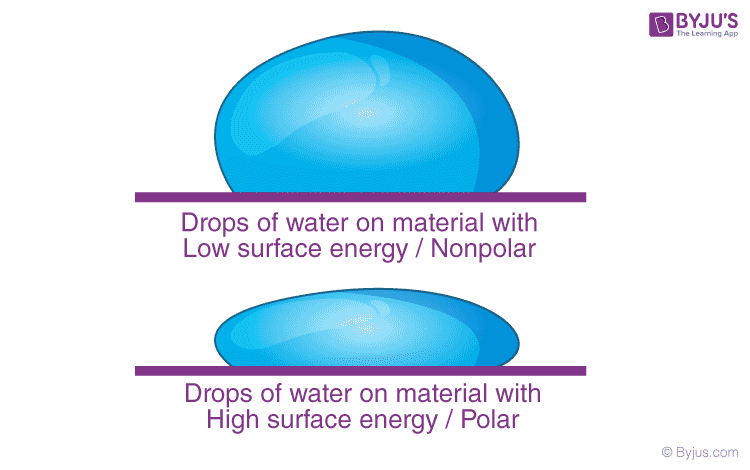# Surface Energy

Surface energy quantifies the disruption of intermolecular bonds that occurs when a surface is created.  It is also called as surface free energy or interfacial free energy. In simple language, surface energy can be defined as the work per unit area done by the force that creates the new surface.

## What is Surface Energy?

Have you ever tried drowning an ant? Doesn’t work, does it? They seem to be body sliding across the water’s surface till they safely reach solid ground. But that doesn’t make sense because their breathing mechanism is kind of located in the underbelly!

They aren’t body sliding. They are just walking across just like how they would do on land except with a different manoeuvring technique. They are able to do this because of the phenomenon known as surface tension. Because of cohesive forces across liquid molecules, surface tension causes the existence of a thin film (layer) across the surface. Surface energy is the work done per unit area to produce this new surface. And if the insect is light enough, to not damage (cause brittle failure to) the layer, it can use the thin layer as support to walk across.### S.I. Unit and Dimension

The SI Unit and dimension of surface energy is given in the table below.

 S.I. Unit N/m Dimension [MT– 2]## Relation Between Surface Energy and Surface Tension (Formula):

 Surface Energy = $$\frac{Energy}{Area}$$

= $$\frac{Joule}{m^{2}}$$

= $$\frac{Newton\times m}{m^{2}}$$

= $$\frac{Newton}{m}$$

= $$\frac{Force}{Length}$$

= Surface Tension

### Excess pressure inside a liquid drop and a bubble:

Consider a small water drop. The drop doesn’t collapse because of the surface tension which is given by:

 σ = F/L

Where,

• σ is the surface tension of the liquid
• F is the force per unit length
• L is the line over which the force acts

If the drop doesn’t collapse, it implies that the pressure within the drop is greater than that outside. The extra pressure (p) is given by subtracting the outside pressure (po) from the inside pressure (pi);

 p = pi – po

Outside Force

= p x surface area of the drop

= (pi – po) x 4πr2

Where,

• r is the radius of the drop

To satisfy the usual tendency for increasing surface area;

 dW = Outside Force = (pi – po) x 4πr2.dr

Work done by the excess pressure is stored in the form of potential energy.

Increase in P.E.

= surface tension × increase in surface area.

= σ x [4π (r+dr2) – 4πr2

= σ x 8πr.dr (after neglecting tiny terms)

dW = Increase in P.E.

This implies that,

(pi – po) x 4πr2.dr = σ x 8πr.dr

(pi – po) = 2σ/r

p = 2σ/r

For a bubble have two free surfaces, like soap bubbles,

 p = 4σ/r

So far we discussed the surface of one liquid. More generally, we might need to consider fluid surface in contact with other fluids or solid surfaces. The surface energy in such cases depends on the materials on both sides of the surface. For example, if the molecules of the materials attract each other, the surface energy is reduced while if they repel each other the surface energy is increased. Thus, more appropriately, the surface energy is the energy of the interface between two materials and depends on both of them.

Stay tuned with BYJU’S to learn more about surface energy and surface tension and other physics concepts with help of interactive video lessons.

Test your knowledge on Surface Energy